Next: Linear restraints Up: Biasing and analysis methods Previous: Metadynamics   Contents   Index

Subsections

## Harmonic restraints

The harmonic biasing method may be used to enforce fixed or moving restraints, including variants of Steered and Targeted MD. Within energy minimization runs, it allows for restrained minimization, e.g. to calculate relaxed potential energy surfaces. In the context of the Colvars module, harmonic potentials are meant according to their textbook definition: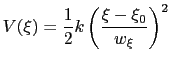(13.24)

Note that this differs from harmonic bond and angle potentials in common force fields, where the factor of one half is typically omitted, resulting in a non-standard definition of the force constant.

The formula above includes the characteristic length scale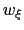of the colvar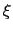(keyword width, see 13.2.1) to allow the definition of a multi-dimensional restraint with a unified force constant:(13.25)

If one-dimensional or homogeneous multi-dimensional restraints are defined, and there are no other uses for the parameter, the parameter width can be left at its default value of.

A harmonic restraint is set up by a harmonic {...} block, which may contain (in addition to the standard option colvars) the following keywords:

• name: see definition of name (biasing and analysis methods)
• colvars: see definition of colvars (biasing and analysis methods)
• outputEnergy: see definition of outputEnergy (biasing and analysis methods)

• forceConstant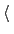Scaled force constant (kcal/mol)Context: harmonic
Acceptable values: positive decimal
Default value: 1.0
Description: This defines a scaled force constant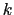for the harmonic potential (eq. 13.26). To ensure consistency for multidimensional restraints, it is divided internally by the square of the specific width for each colvar involved (which is 1 by default), so that all colvars are effectively dimensionless and of commensurate size. For instance, setting a scaled force constant of 10 kcal/mol acting on two colvars, an angle with a width of 5 degrees and a distance with a width of 0.5 Å, will apply actual force constants of 0.4 kcal/moldegree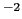for the angle and 40 kcal/mol/Åfor the distance.

• centersInitial harmonic restraint centersContext: harmonic
Acceptable values: space-separated list of colvar values
Description: The centers (equilibrium values) of the restraint,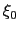, are entered here. The number of values must be the number of requested colvars. Each value is a decimal number if the corresponding colvar returns a scalar, a (x, y, z)'' triplet if it returns a unit vector or a vector, and a (q0, q1, q2, q3)'' quadruplet if it returns a rotational quaternion. If a colvar has periodicities or symmetries, its closest image to the restraint center is considered when calculating the harmonic potential.

Tip: A complex set of restraints can be applied to a system, by defining several colvars, and applying one or more harmonic restraints to different groups of colvars. In some cases, dozens of colvars can be defined, but their value may not be relevant: to limit the size of the colvars trajectory file, it may be wise to disable outputValue for such ancillary'' variables, and leave it enabled only for relevant'' ones.

### Moving restraints: steered molecular dynamics

The following options allow to change gradually the centers of the harmonic restraints during a simulations. When the centers are changed continuously, a steered MD in a collective variable space is carried out.

• targetCentersSteer the restraint centers towards these targetsContext: harmonic
Acceptable values: space-separated list of colvar values
Description: When defined, the current centers will be moved towards these values during the simulation. By default, the centers are moved over a total of targetNumSteps steps by a linear interpolation, in the spirit of Steered MD. If targetNumStages is set to a nonzero value, the change is performed in discrete stages, lasting targetNumSteps steps each. This second mode may be used to sample successive windows in the context of an Umbrella Sampling simulation. When continuing a simulation run, the centers specified in the configuration filecolvarsConfig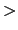are overridden by those saved in the restart filecolvarsInput. To perform Steered MD in an arbitrary space of colvars, it is sufficient to use this option and enable outputAppliedForce within each of the colvars involved.

• targetNumStepsNumber of steps for steeringContext: harmonic
Acceptable values: positive integer
Description: In single-stage (continuous) transformations, defines the number of MD steps required to move the restraint centers (or force constant) towards the values specified with targetCenters or targetForceConstant. After the target values have been reached, the centers (resp. force constant) are kept fixed. In multi-stage transformations, this sets the number of MD steps per stage.

• outputCentersWrite the current centers to the trajectory fileContext: harmonic
Acceptable values: boolean
Default value: off
Description: If this option is chosen and colvarsTrajFrequency is not zero, the positions of the restraint centers will be written to the trajectory file during the simulation. This option allows to conveniently extract the PMF from the colvars trajectory files in a steered MD calculation.

• outputAccumulatedWorkWrite the accumulated work of the moving restraint to the trajectory fileContext: harmonic
Acceptable values: boolean
Default value: off
Description: If this option is chosen, targetCenters is defined, and colvarsTrajFrequency is not zero, the accumulated work from the beginning of the simulation will be written to the trajectory file. If the simulation has been continued from a previous state file, the previously accumulated work is included in the integral. This option allows to conveniently extract the PMF from the colvars trajectory files in a steered MD calculation.

Note on restarting moving restraint simulations: Information about the current step and stage of a simulation with moving restraints is stored in the restart file (state file). Thus, such simulations can be run in several chunks, and restarted directly using the same colvars configuration file. In case of a restart, the values of parameters such as targetCenters, targetNumSteps, etc. should not be changed manually.

### Moving restraints: umbrella sampling

The centers of the harmonic restraints can also be changed in discrete stages: in this cases a one-dimensional umbrella sampling simulation is performed. The sampling windows in simulation are calculated in sequence. The colvars trajectory file may then be used both to evaluate the correlation times between consecutive windows, and to calculate the frequency distribution of the colvar of interest in each window. Furthermore, frequency distributions on a predefined grid can be automatically obtained by using the histogram bias (see 13.5.7).

To activate an umbrella sampling simulation, the same keywords as in the previous section can be used, with the addition of the following:

• targetNumStagesNumber of stages for steeringContext: harmonic
Acceptable values: non-negative integer
Default value: 0
Description: If non-zero, sets the number of stages in which the restraint centers or force constant are changed to their target values. If zero, the change is continuous. Each stage lasts targetNumSteps MD steps. To sample both ends of the transformation, the simulation should be run for targetNumSteps(targetNumStages + 1).

### Changing force constant

The force constant of the harmonic restraint may also be changed to equilibrate .

• targetForceConstantChange the force constant towards this valueContext: harmonic
Acceptable values: positive decimal
Description: When defined, the current forceConstant will be moved towards this value during the simulation. Time evolution of the force constant is dictated by the targetForceExponent parameter (see below). By default, the force constant is changed smoothly over a total of targetNumSteps steps. This is useful to introduce or remove restraints in a progressive manner. If targetNumStages is set to a nonzero value, the change is performed in discrete stages, lasting targetNumSteps steps each. This second mode may be used to compute the conformational free energy change associated with the restraint, within the FEP or TI formalisms. For convenience, the code provides an estimate of the free energy derivative for use in TI. A more complete free energy calculation (particularly with regard to convergence analysis), while not handled by the Colvars module, can be performed by post-processing the colvars trajectory, if colvarsTrajFrequency is set to a suitably small value. It should be noted, however, that restraint free energy calculations may be handled more efficiently by an indirect route, through the determination of a PMF for the restrained coordinate.

• targetForceExponentExponent in the time-dependence of the force constantContext: harmonic
Acceptable values: decimal equal to or greater than 1.0
Default value: 1.0
Description: Sets the exponent,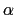, in the function used to vary the force constant as a function of time. The force is varied according to a coupling parameter, raised to the power: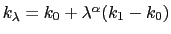, where,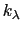, and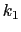are the initial, current, and final values of the force constant. The parameterevolves linearly from 0 to 1, either smoothly, or in targetNumStages equally spaced discrete stages, or according to an arbitrary schedule set with lambdaSchedule. When the initial value of the force constant is zero, an exponent greater than 1.0 distributes the effects of introducing the restraint more smoothly over time than a linear dependence, and ensures that there is no singularity in the derivative of the restraint free energy with respect to lambda. A value of 4 has been found to give good results in some tests.

• targetEquilStepsNumber of steps discarded from TI estimateContext: harmonic
Acceptable values: positive integer
Description: Defines the number of steps within each stage that are considered equilibration and discarded from the restraint free energy derivative estimate reported reported in the output.

• lambdaScheduleSchedule of lambda-points for changing force constantContext: harmonic
Acceptable values: list of real numbers between 0 and 1
Description: If specified together with targetForceConstant, sets the sequence of discretevalues that will be used for different stages.Next: Linear restraints Up: Biasing and analysis methods Previous: Metadynamics   Contents   Index
vmd@ks.uiuc.edu